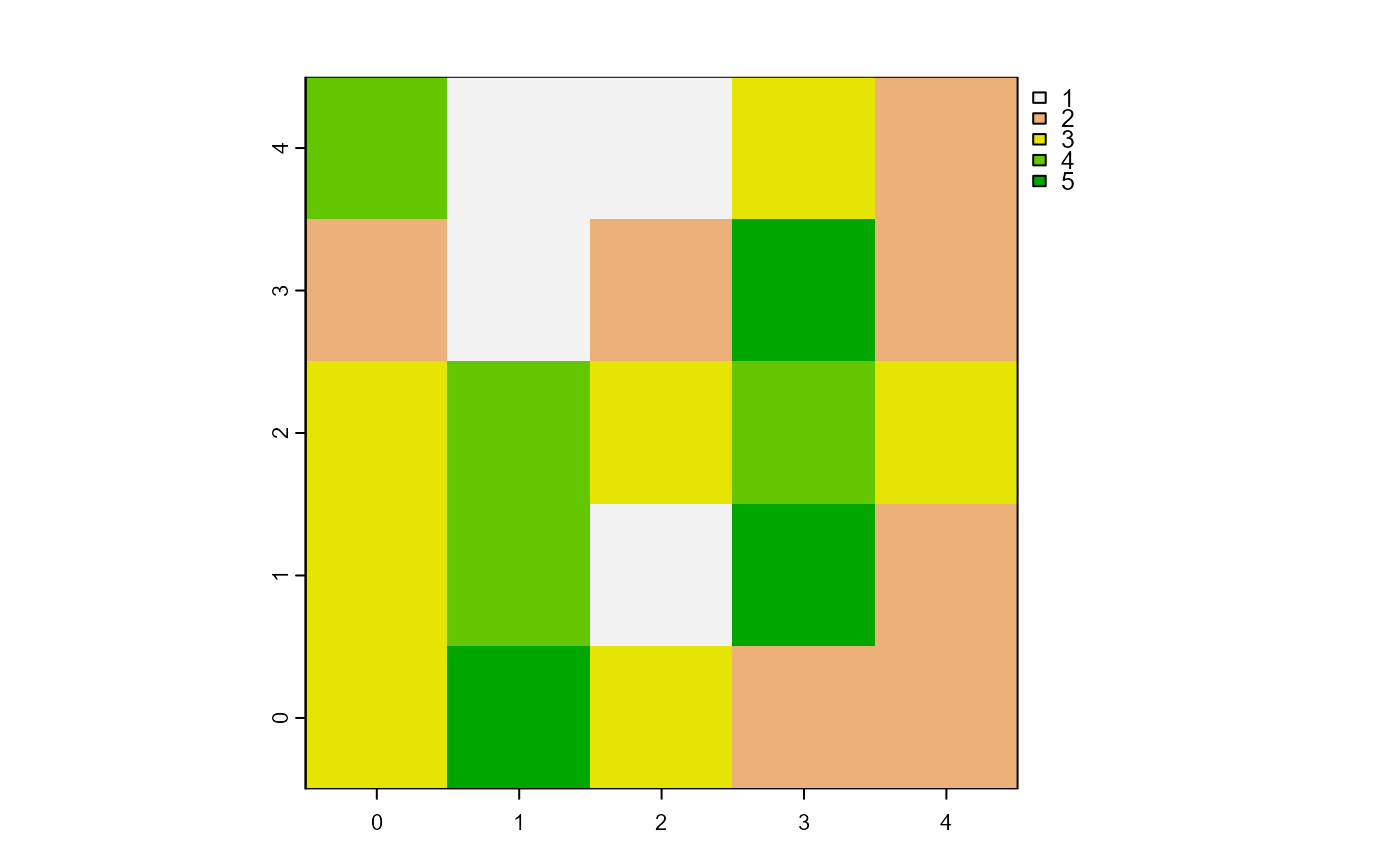Report one patch or one turtle among agents which has its variable equals to the minimum value.

minOneOf(agents, world, var)

# S4 method for matrix,worldMatrix,missing
minOneOf(agents, world)

# S4 method for matrix,worldArray,character
minOneOf(agents, world, var)

# S4 method for agentMatrix,missing,character
minOneOf(agents, var)

## Arguments

agents

Matrix (ncol = 2) with the first column pxcor and the second column pycor representing the patches coordinates, or

          AgentMatrix object representing the moving agents.

world

WorldMatrix or worldArray object.

var

Character. The name of the selected agents variable. If agents are patches and the world is a worldMatrix object, var must not be provided. If agents are patches and the world is a worldArray object, var is the name of the layer to use to define the patches values. If agents are turtles, var is one of the turtles' variable and can be equal to xcor, ycor, any of the variables created when turtles were created, as well as any variable created using turtlesOwn().

## Value

Matrix (ncol = 2, nrow = 1) with the first column pxcor and the second column pycor representing the coordinates of the patch

(or of one of the patches) among the agents which has its variable equals to the minimum value among the agents, or

    AgentMatrix of length 1 representing the turtle (or one of

the turtles) among the agents which has its variable var

equals to the minimum value

among the agents.

## Details

world must not be provided if agents are turtles.

     If there are several patches or turtles among agents with their

variable equal to the minimum

value, one is chosen randomly. To access to all patches or turtles among

agents which have their variable equal

to the minimum value, use withMin().

Sarah Bauduin

## Examples

# Patches
w1 <- createWorld(
minPxcor = 0, maxPxcor = 4, minPycor = 0, maxPycor = 4,
data = sample(1:5, size = 25, replace = TRUE)
)
plot(w1)p1 <- minOneOf(agents = patches(w1), world = w1)

# Turtles
t1 <- createTurtles(
n = 10, coords = randomXYcor(w1, n = 10),
heading = sample(1:3, size = 10, replace = TRUE)
)
t2 <- minOneOf(agents = t1, var = "heading")